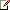Anova1Way Method

Measurement Studio 2012 for Visual Studio 2010 Help

Edition Date: August 2012

Part Number: 372636F-01

»View Product InfoDownload Help (Windows Only)Note: This topic applies to the following Measurement Studio editions: Enterprise.

Performs a one-way analysis of variance in the fixed-effect model.

Namespace: NationalInstruments.Analysis.Math

Assembly: NationalInstruments.Analysis.Enterprise (in NationalInstruments.Analysis.Enterprise.dll) Version: 12.0.40.318

Syntax

Visual Basic (Declaration)
<SecurityPermissionAttribute(SecurityAction.Demand, Flags := SecurityPermissionFlag.UnmanagedCode)> _
Public Shared Function Anova1Way ( _
observations As Double(), _
levels As Integer(), _
numberOfLevels As Integer _
) As Statistics.Anova1WayOutput

C#
[SecurityPermissionAttribute(SecurityAction.Demand, Flags = SecurityPermissionFlag.UnmanagedCode)]
public static Statistics.Anova1WayOutput Anova1Way(
double[] observations,
int[] levels,
int numberOfLevels
)

Parameters

observations
Type: System.Double []
The experimental observations.
levels
Type: System.Int32 []
The level to which the corresponding observation belongs.
numberOfLevels
Type: System.Int32
The total number of different levels that are assumed by the experimental factor.

Return Value

The output of the one-way analysis of variance. This is specified using Statistics.Anova1WayOutput.

Exceptions

ExceptionCondition
ArgumentException

The number of samples in observations is equal to zero.

-or-

levels has missing observations.

-or-

The number of samples in levels is less than one or greater than or equal to the number of elements in observations.

ArgumentNullException

observations is null (Nothing in Visual Basic).

-or-

levels is null (Nothing in Visual Basic).

DllNotFoundException The analysis library cannot be found.
EntryPointNotFoundException A required operation in the analysis library cannot be found.
InvalidOperationException Level of factor is outside the allowable range.
OutOfMemoryException There is not enough memory to carry out this operation.

Remarks

Anova1Way takes an array, X, of experimental observations made at various levels of a factor, with at least one observation per level, and performs a one-way analysis of variance in the fixed-effect model. In the one-way analysis of variance, the method tests whether the level of the factor has an effect on the experimental outcome.

Refer to Factors and Levels, Statistical Model, Assumptions, Hypothesis, General Method, Testing the Hypothesis, and Formulas for more information.

Examples

The following example tests the hypothesis that the amount of rainfall does not affect the crop output. This means that every level has the same mean for the crop output. The array, observations, indicates the crop output and the array, rain, indicates the amount of rainfall in inches. The output of the one-way analysis of variance is stored in the variable, output. If output.Significance is too large, we should reject our hypothesis. This means the amount of rainfall does affect the crop output.
VB.NET
Dim numSamples As Integer = 9
Dim observations() As Double = {123.0, 122.0, 126.0, 124.0, 140.0, 141.0, 143.0, 120.0, 118.0, 128.0}
Dim rain() As Double = {8.0, 1.0, 1.5, 0.5, 2.5, 3.5, 3.0, 6.0, 8.0, 0.0}
Dim levels(numSamples) As Integer
Dim numberOfLevels As Integer = 0
Dim output As Statistics.Anova1WayOutput

For i As Integer = 1 To rain.Length - 1
If (rain(i) <= 2.0) Then
levels(i) = 0
ElseIf (rain(i) <entity value="gt" /> 4.0) Then
levels(i) = 2
Else
levels(i) = 1
End If
Next

For i As Integer = 1 To levels.Length - 1
If (numberOfLevels < levels(i)) Then
numberOfLevels = levels(i)
End If
Next

' Total number of different levels
numberOfLevels = numberOfLevels + 1

' Perform one-way analysis of variance on array, observations. The array consists of experimental observations made at various levels of a factor, with at least one observation per level.
output = Statistics.Anova1Way(observations, levels, numberOfLevels)
C#
int numSamples = 10;
double[] observations = new double[] { 123.0, 122.0, 126.0, 124.0, 140.0, 141.0, 143.0, 120.0, 118.0, 128.0 };
double[] rain = new double[] { 8.0, 1.0, 1.5, 0.5, 2.5, 3.5, 3.0, 6.0, 8.0, 0.0 };
int[] levels = new int[numSamples];
int numberOfLevels = 0;
Statistics.Anova1WayOutput output = new Statistics.Anova1WayOutput();

for (int i = 0; i < rain.Length; i++)
{
if (rain[i] <= 2.0)
levels[i] = 0;
else if (rain[i] <entity value="gt" /> 4.0)
levels[i] = 2;
else
levels[i] = 1;
}

for (int i = 0; i < levels.Length; i++)
{
if (numberOfLevels < levels[i])
numberOfLevels = levels[i];
}

// Total number of different levels
numberOfLevels++;

// Perform one-way analysis of variance on array, observations. The array consists of experimental observations made at various levels of a factor, with at least one observation per level.
output = Statistics.Anova1Way(observations, levels, numberOfLevels);